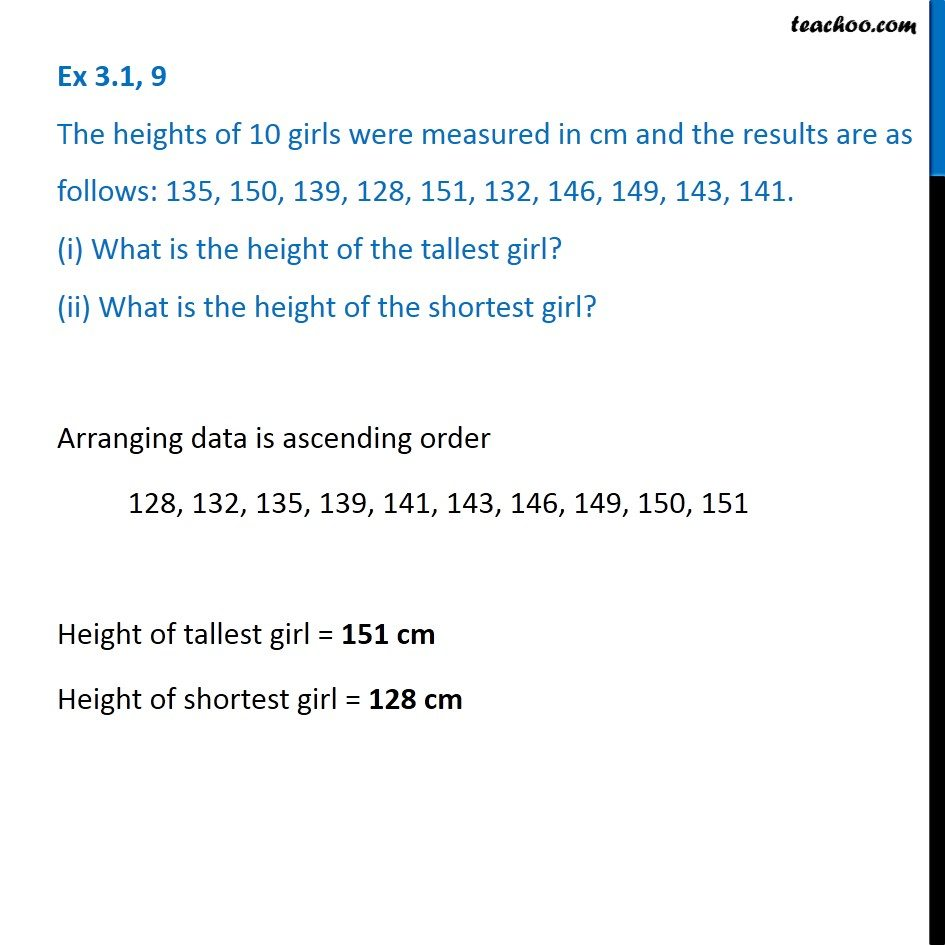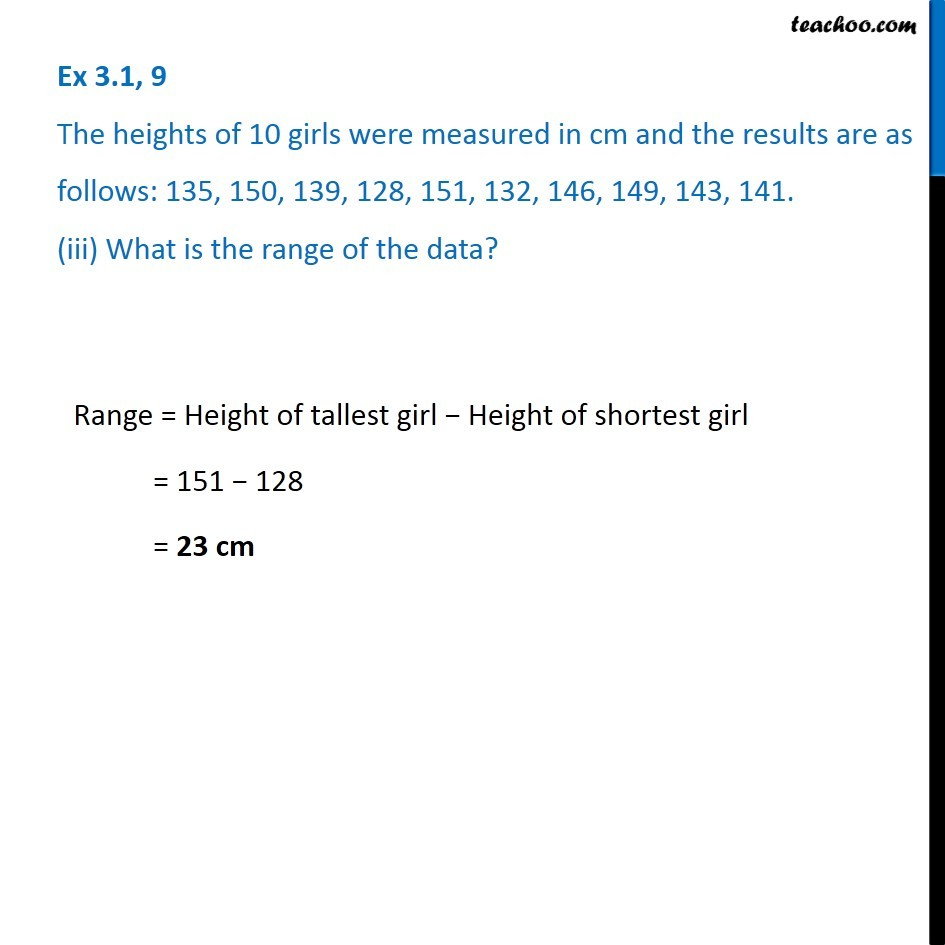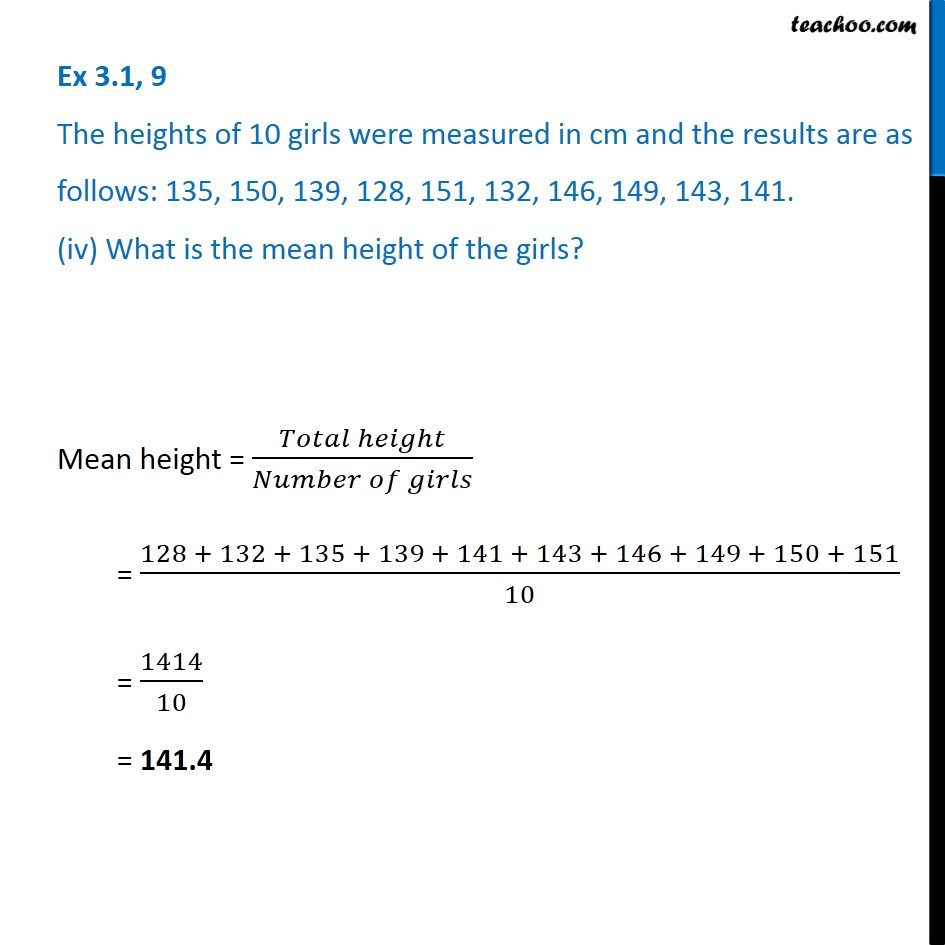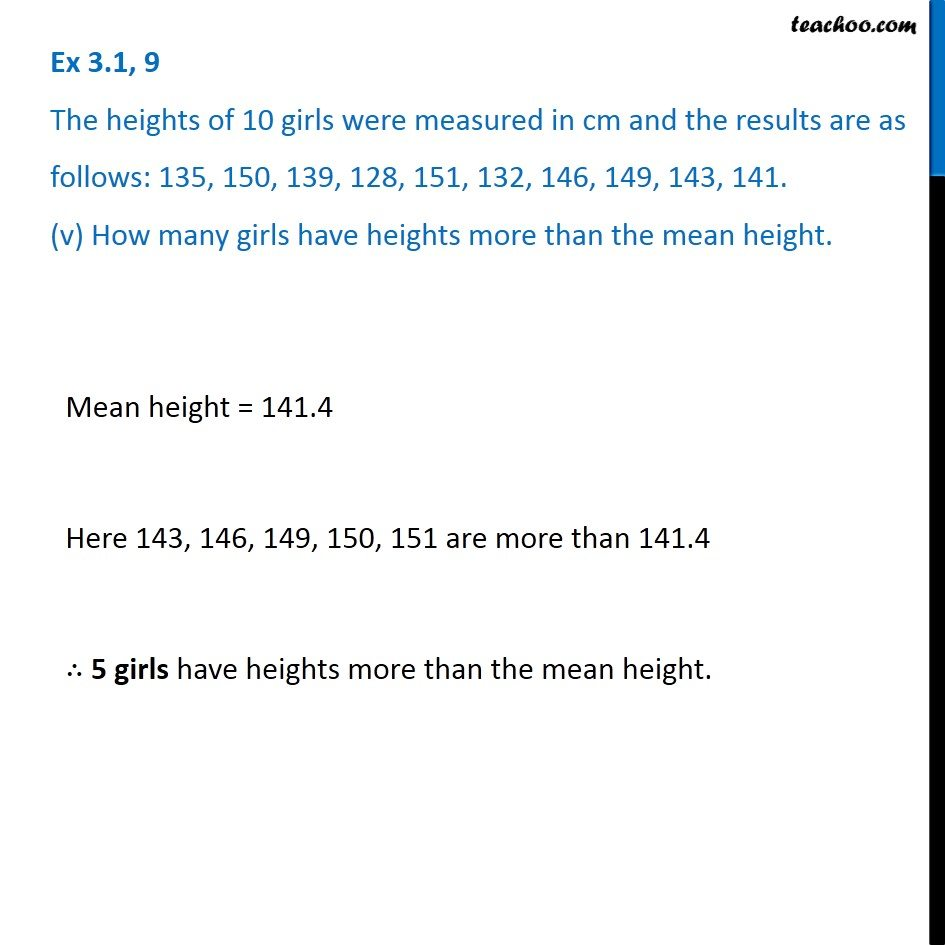Ex 3.1

Chapter 3 Class 7 Data Handling
Serial order wise### Transcript

Ex 3.1, 9 The heights of 10 girls were measured in cm and the results are as follows: 135, 150, 139, 128, 151, 132, 146, 149, 143, 141. (i) What is the height of the tallest girl? (ii) What is the height of the shortest girl? Arranging data is ascending order 128, 132, 135, 139, 141, 143, 146, 149, 150, 151 Height of tallest girl = 151 cm Height of shortest girl = 128 cm Ex 3.1, 9 The heights of 10 girls were measured in cm and the results are as follows: 135, 150, 139, 128, 151, 132, 146, 149, 143, 141. (iii) What is the range of the data? Range = Height of tallest girl − Height of shortest girl = 151 − 128 = 23 cm Ex 3.1, 9 The heights of 10 girls were measured in cm and the results are as follows: 135, 150, 139, 128, 151, 132, 146, 149, 143, 141. (iv) What is the mean height of the girls? Mean height = (𝑇𝑜𝑡𝑎𝑙 ℎ𝑒𝑖𝑔ℎ𝑡)/(𝑁𝑢𝑚𝑏𝑒𝑟 𝑜𝑓 𝑔𝑖𝑟𝑙𝑠) = (128 + 132 + 135 + 139 + 141 + 143 + 146 + 149 + 150 + 151)/10 = 1414/10 = 141.4 Ex 3.1, 9 The heights of 10 girls were measured in cm and the results are as follows: 135, 150, 139, 128, 151, 132, 146, 149, 143, 141. (v) How many girls have heights more than the mean height. Mean height = 141.4 Here 143, 146, 149, 150, 151 are more than 141.4 ∴ 5 girls have heights more than the mean height.